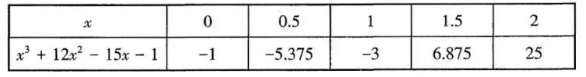$\text{A.}$ $0 < x < 0.5$ $\text{B.}$ $0.5 < x < 1$ $\text{C.}$ $1 < x < 1.5$ $\text{D.}$ $1.5 < x < 2$
【答案】 C